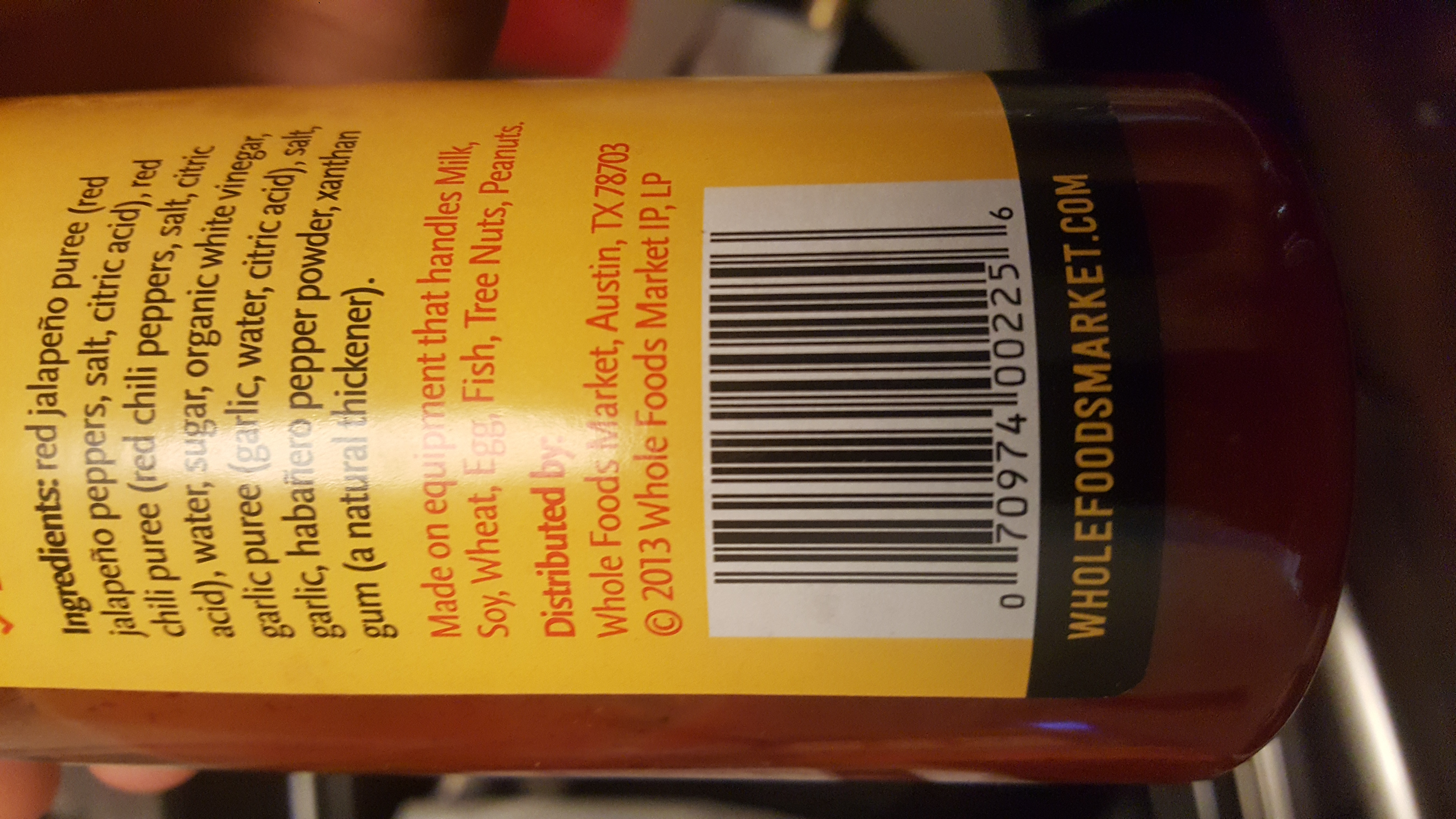{{ message }}

Instantly share code, notes, and snippets.

# gyurisc/detect_barcode.py

Created Nov 20, 2015
Detecting Barcodes in Images with Python and OpenCV
 # Detecting Barcodes in Images with Python and OpenCV # OpenCV 3.0 # http://www.pyimagesearch.com/2014/11/24/detecting-barcodes-images-python-opencv/ import numpy as np import argparse import cv2 ap = argparse.ArgumentParser() ap.add_argument("-i","--image", required = True, help="path to image file") args = vars(ap.parse_args()) #load the image and convert it to grayscale image = cv2.imread(args["image"]) gray = cv2.cvtColor(image, cv2.COLOR_BGR2GRAY) # compute the Scharr gradient magnitude reprtesentation of the images # in both the x and y direction gradX = cv2.Sobel(gray, ddepth = cv2.CV_32F, dx = 1, dy = 0, ksize = -1) gradY = cv2.Sobel(gray, ddepth = cv2.CV_32F, dx = 0, dy = 1, ksize = -1) #substract the y-gradient from the x-gradient gradient = cv2.subtract(gradX, gradY) gradient = cv2.convertScaleAbs(gradient) #blur and threshold the image blurred = cv2.blur(gradient, (9,9)) (_, thresh) = cv2.threshold(blurred, 225, 255, cv2.THRESH_BINARY) # construct a closing kernel and apply it to the thresholded image kernel = cv2.getStructuringElement(cv2.MORPH_RECT, (21, 7)) closed = cv2.morphologyEx(thresh, cv2.MORPH_CLOSE, kernel) # perform a series of erosions and dilations closed = cv2.erode(closed, None, iterations = 4) closed = cv2.dilate(closed, None, iterations = 4) # find the contours in the thresholded image, then sort the contours # by their area, keeping only the largest one (_, cnts, _) = cv2.findContours(closed.copy(), cv2.RETR_EXTERNAL, cv2.CHAIN_APPROX_SIMPLE) c = sorted(cnts, key = cv2.contourArea, reverse = True) # compute the rotated bounding box of the largest contour rect = cv2.minAreaRect(c) box = np.int0(cv2.boxPoints(rect)) # draw a bounding box arounded the detected barcode and display the # image cv2.drawContours(image, [box], -1, (0, 255, 0), 2) #cv2.imshow("Gradient", gradient) #cv2.imshow("Blurred", blurred) #cv2.imshow("Threshold", thresh) #cv2.imshow("Kernel", kernel) #cv2.imshow("Closing", closed) cv2.imshow("Image", image) cv2.waitKey(5000)

###gyurisc commented Nov 20, 2015

 Following the article and fixed corrected some issues that arised using a newer version of OpenCV

###bdonnahue commented Jan 3, 2018

 @gyurisc I am having a problem getting this to work with a barcode I have attached. Any advice would be great!